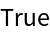Function Repository Resource:

Perform a folding operation parsing multiple arguments

Contributed by: Sander Huisman

Examples

Basic Examples

Basic example:

 In:=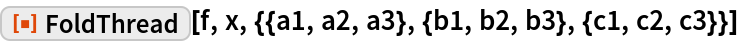Out=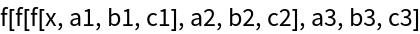Scope

Without an initializer, the first element of each list is the initializer:

 In:=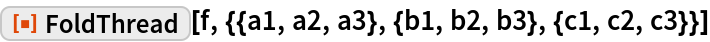Out=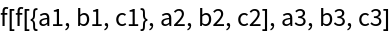Make an operator:

 In:=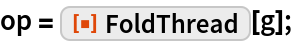Feed a list of lists:

 In:=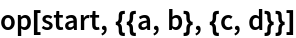Out=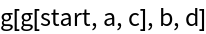Applications

Use Integrate to integrate x from 0 to 2, y from 0 to 2 and z from 0 to 2:

 In:=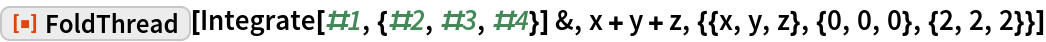Out=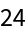Create a continued fraction with different numerators:

 In:=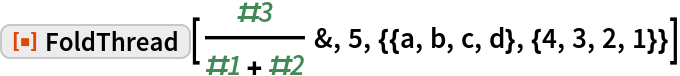Out=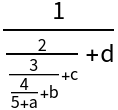Properties and Relations

Fold and FoldThread give equivalent results for the case in which a single argument is folded:

 In:=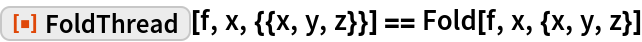Out=